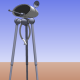## GIRC Path CalculationsThe user guides the robot in GIRC by creating a path. The path is composed of segments which are created by mouse clicks. The program calculates the length (or distance traveled) for the path segment and also the heading.

“Heading relative to what?” you ask, and that’s a good question. I assumed that the positive x-axis would be zero degrees and that the angle would increase going counterclockwise. Instead, it appears in the display that the angles are calculated going clockwise from the positive x-axis. I don’t know if that’s a property of Processing’s trig functions or if I did the formulas wrong. I’ll find out.

Anyhow, the distance looks right.

(NOTE: The TURN value is currently showing the segment number.)